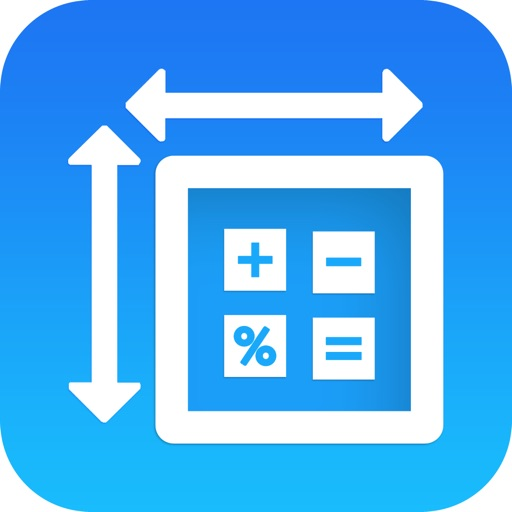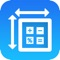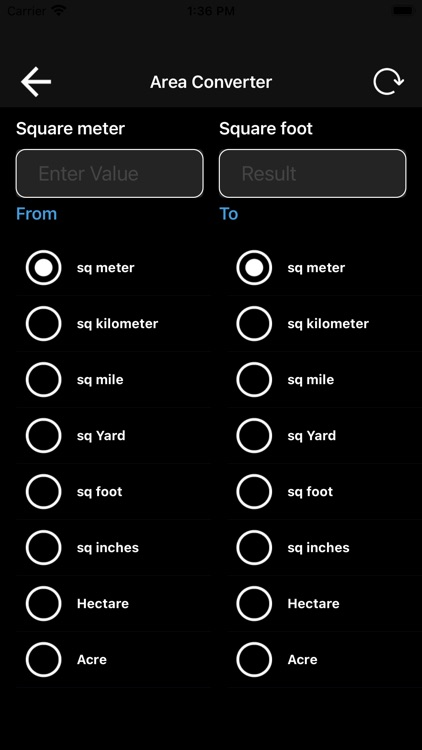## Application to calculate an area of a room# Square meters calculator

by DOBARIYA SMITApplication to calculate an area of a room. It should have a square or rectangular shape. You can also calculate an area of a space (cuboid or cubic shape).Version
1.1
Rating
NA
Size
13Mb
Genre
Utilities
Last updated
May 31, 2022
Release date
May 9, 2022

### App Screenshots### App Store Description

Application to calculate an area of a room. It should have a square or rectangular shape. You can also calculate an area of a space (cuboid or cubic shape).

You just have to set the width, height in feet and inches, and the area will be displayed instantly. Height should also be set to calculate the volume.

You can add the price per square or cubic feet or inches and the total price will be also added.

Square meter app: Square meter app to Calculate Square meter, Cubic Meter, total square meter, total cubic meter.
Calculate square feet and inches as well as cubic feet and inches.

** How to Calculate Square Meters:

You might find yourself needing the area of a room or other space in square meters for a flooring, renovation, or landscaping project.
You can measure the area of a space in square meters in a few simple steps.

>> Follow along to learn how to calculate yourself, or simply use the calculator above:

1 > : Measure Length and Width
Start by measuring the length and width of the space in meters using a tape measure or other measuring tool.

2 > : If your measurement is in another unit of measure, centimeters for example, then you’ll need to convert to square meters.
We have some simple area conversion utilities, or you can convert yourself. If your measurement is in centimeters then divide by 100.

3 > : Multiply Measurements Together
Now that you have a length and width measurement, you can find the area in square meters by multiplying the length and width together.

square meters = length × width

**How to calculate cubic meters :

If your unit of measurement is not meter, convert the unit to meter first, then, multiply length, width and height values together, this will give you the volume of the cube.

** Price Per Square Foot Calculator :

Use this price per square foot calculator to work out the cost price per square foot of floor space for purchases, lease prices, and rentals on property.

Length Converter Following Units:

> Meter

> Centimeter

> Millimetre

> Micrometre

> Mile

> Yard

> Foot

> Inch

Area Converter Following Units:

> Square meter

> Square kilometer

> Square mile

> Square yard

> Square foot

> Square inch

Disclaimer:
AppAdvice does not own this application and only provides images and links contained in the iTunes Search API, to help our users find the best apps to download. If you are the developer of this app and would like your information removed, please send a request to takedown@appadvice.com and your information will be removed.